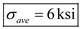# For the given stale of stress, determine (a) the orientation of the planes of maximum in-plane shearing stress, (b) the maximum in-plane shearing stress, (c) the corresponding normal stress.

Question-AnswerCategory: Strength of MaterialsFor the given stale of stress, determine (a) the orientation of the planes of maximum in-plane shearing stress, (b) the maximum in-plane shearing stress, (c) the corresponding normal stress.

For the given stale of stress, determine (a) the orientation of the planes of maximum in-plane shearing stress, (b) the maximum in-plane shearing stress, (c) the corresponding normal stress.Step: 1

Schemaic diagram of element: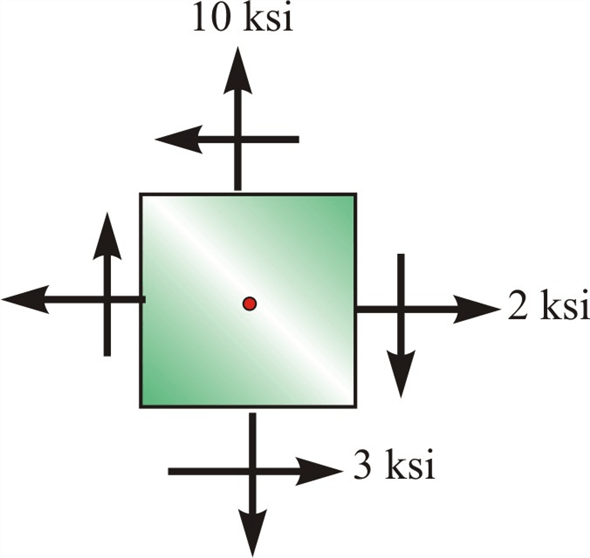Step: 2

Compressive stress in x-direction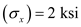Compressive stress in y-direction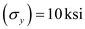Shear stress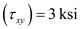a) The orientation of the planes of maximum in-plane shearing stress
Maximum planes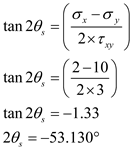Therefore, principal planes is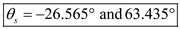Step: 3

b)
Maximum in plane shear stress is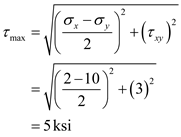Therefore, maximum shear stress isStep: 4

c) Normal stress isTherefore, normal stress is